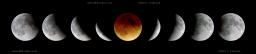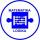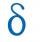# Sequence

Write the first 6 members of this sequence:

a1 = 5
a2 = 7
an+2 = an+1 +2 an

a1 =  5
a2 =  7
a3 =  17
a4 =  31
a5 =  65
a6 =  127

### Step-by-step explanation:

${a}_{1}=5$
${a}_{2}=7$
${a}_{3}=7+2\cdot 5=17$
${a}_{4}=17+2\cdot 7=31$
${a}_{5}=31+2\cdot 17=65$
${a}_{6}=65+2\cdot 31=127$Did you find an error or inaccuracy? Feel free to write us. Thank you!## Related math problems and questions:

• SequenceWrite the first 7 members of an arithmetic sequence: a1=-3, d=6.
• Five membersWrite first 5 members geometric sequence and determine whether it is increasing or decreasing: a1 = 3 q = -2
• AP 6Calculate the first five items of an arithmetic sequence if it is given: a2 – a3 + a5 = 20 a1 + a6 = 38
• Sequence 2Write the first 5 members of an arithmetic sequence a11=-14, d=-1
• Sequence 3Write the first 5 members of an arithmetic sequence: a4=-35, a11=-105.
• Sequence - 5 membersWrite first five members of the sequence ?
• Geometric sequence 5About members of geometric sequence we know: ? ? Calculate a1 (first member) and q (common ratio or q-coefficient)
• SequenceIn the arithmetic sequence is a1=-1, d=4. Which member is equal to the number 203?
• SequenceIn the arithmetic sequence is given: Sn=2304, d=2, an=95 Calculate a1 and n.
• Geometric sequence 3In geometric sequence is a8 = 312500; a11= 39062500; sn=1953124. Calculate the first item a1, quotient q, and n - number of members by their sum s_n.
• AS - sequenceWhat are the first ten members of the sequence if a11=22, d=2.
• SequenceBetween numbers 1 and 53 insert n members of the arithmetic sequence that its sum is 702.
• AP - simpleFind the first ten members of the sequence if a11 = 132, d = 3.
• Finite arithmetic sequenceHow many numbers should be inserted between the numbers 1 and 25 so that all numbers create a finite arithmetic sequence and that the sum of all members of this group is 117?
• Difference APCalculate the difference of arithmetic progression if the sum of its first 19 members Sn = 8075 and the first member is a1 = 20
• GP membersThe geometric sequence has 10 members. The last two members are 2 and -1. Which member is -1/16?
• Geometric sequence 4It is given geometric sequence a3 = 7 and a12 = 3. Calculate s23 (= sum of the first 23 members of the sequence).